# Chapter 10 Self Test

## Calculating Fractional Coverage

If KCO=1 atm-1 amd KNO=5 atm-1, what is the ratio of sites containing CO to those containing NO when the conversion is 20%? 99%? What fraction of sites are occupied by NO? by CO? The feed is equal molar in CO and NO with PO=2 atm.

If KCO=1 atm-1 amd KNO=5 atm-1, what is the ratio of sites containing CO to those containing NO when the conversion is 20%? 99%? What fraction of sites are occupied by NO? by CO? The feed is equal molar in CO and NO with PO=2 atm.

Solution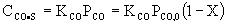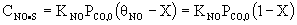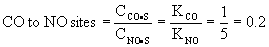Fraction of CO sites.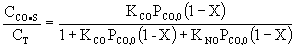For X=.2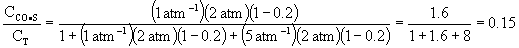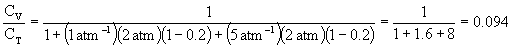at 20% conversion 15% of the sites are occupied by CO and 9.4% are vacant.

For X=.99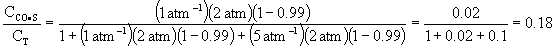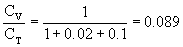at 99% conversion 1.8% of the sites are occupied by CO and 89% are vacant.

Back to Chapter 10